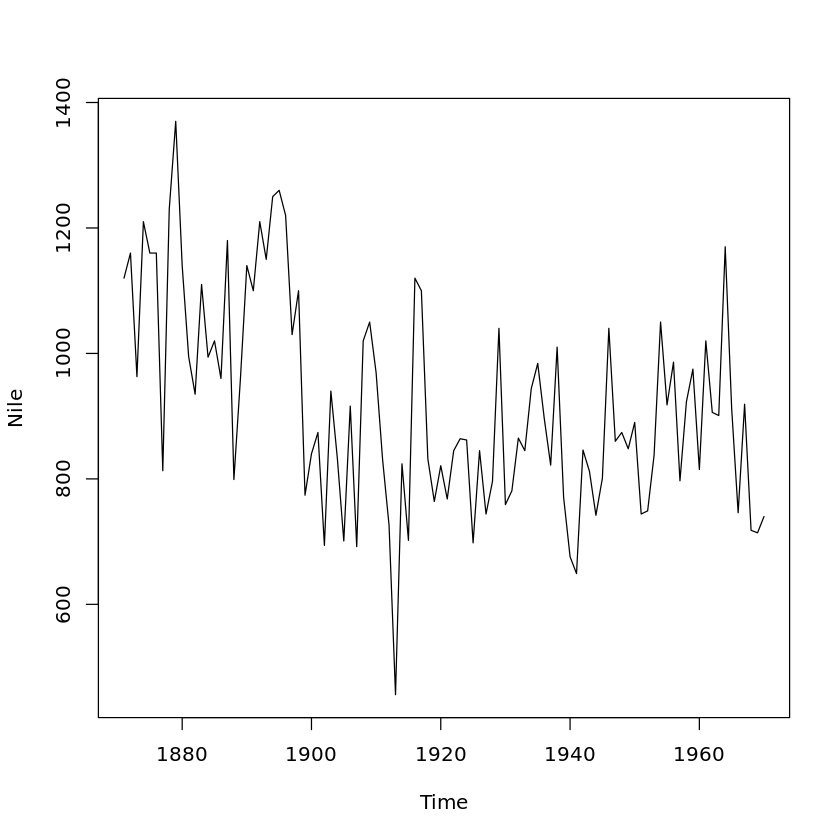# Stub 2 (Using R)

$$\newcommand{\vecs}{\overset { \rightharpoonup} {\mathbf{#1}} }$$ $$\newcommand{\vecd}{\overset{-\!-\!\rightharpoonup}{\vphantom{a}\smash {#1}}}$$$$\newcommand{\id}{\mathrm{id}}$$ $$\newcommand{\Span}{\mathrm{span}}$$ $$\newcommand{\kernel}{\mathrm{null}\,}$$ $$\newcommand{\range}{\mathrm{range}\,}$$ $$\newcommand{\RealPart}{\mathrm{Re}}$$ $$\newcommand{\ImaginaryPart}{\mathrm{Im}}$$ $$\newcommand{\Argument}{\mathrm{Arg}}$$ $$\newcommand{\norm}{\| #1 \|}$$ $$\newcommand{\inner}{\langle #1, #2 \rangle}$$ $$\newcommand{\Span}{\mathrm{span}}$$ $$\newcommand{\id}{\mathrm{id}}$$ $$\newcommand{\Span}{\mathrm{span}}$$ $$\newcommand{\kernel}{\mathrm{null}\,}$$ $$\newcommand{\range}{\mathrm{range}\,}$$ $$\newcommand{\RealPart}{\mathrm{Re}}$$ $$\newcommand{\ImaginaryPart}{\mathrm{Im}}$$ $$\newcommand{\Argument}{\mathrm{Arg}}$$ $$\newcommand{\norm}{\| #1 \|}$$ $$\newcommand{\inner}{\langle #1, #2 \rangle}$$ $$\newcommand{\Span}{\mathrm{span}}$$$$\newcommand{\AA}{\unicode[.8,0]{x212B}}$$

R Session: NOT STARTED

Query $$\PageIndex{1}$$

(unable to fetch text document from uri [status: 403 (Forbidden)])
print('hello world')
 "hello world"


Original text created by N. Matloff.

print('somthing test')
Hello world!


## Lesson 1: First R Steps

The R command prompt is '>'. Again, it will be shown here, but you don't type it. It is just there in your R window to let you know R is inviting you to submit a command. (If you are using RStudio, you'll see it in the Console pane.) So, just type '1+1' then hit Enter. Sure enough, it prints out 2 (you were expecting maybe 12108?):

$E =mc^2 \label{Einstein}$ See equation \ref{Einstein}

But what is that '' here? It's just a row label. We'll go into that later, not needed quite yet. R includes a number of built-in datasets, mainly for illustration purposes. One of them is Nile, 100 years of annual flow data on the Nile River. Let's find the mean flow:

mean(Nile) + 10
929.35

Here mean is an example of an R function, and in this case Nile is an argument -- fancy way of saying "input" -- to that function. That output, 919.35, is called the return value or simply value. The act of running the function is termed calling the function.

Another point to note is that we didn't need to call R's print function. We could have typed,

print(mean(Nile))

but whenever we are at the R '>' prompt, any expression we type will be printed out.

Since there are only 100 data points here, it's not unwieldy to print them out:

Nile
A Time Series:
1. 1120
2. 1160
3. 963
4. 1210
5. 1160
6. 1160
7. 813
8. 1230
9. 1370
10. 1140
11. 995
12. 935
13. 1110
14. 994
15. 1020
16. 960
17. 1180
18. 799
19. 958
20. 1140
21. 1100
22. 1210
23. 1150
24. 1250
25. 1260
26. 1220
27. 1030
28. 1100
29. 774
30. 840
31. 874
32. 694
33. 940
34. 833
35. 701
36. 916
37. 692
38. 1020
39. 1050
40. 969
41. 831
42. 726
43. 456
44. 824
45. 702
46. 1120
47. 1100
48. 832
49. 764
50. 821
51. 768
52. 845
53. 864
54. 862
55. 698
56. 845
57. 744
58. 796
59. 1040
60. 759
61. 781
62. 865
63. 845
64. 944
65. 984
66. 897
67. 822
68. 1010
69. 771
70. 676
71. 649
72. 846
73. 812
74. 742
75. 801
76. 1040
77. 860
78. 874
79. 848
80. 890
81. 744
82. 749
83. 838
84. 1050
85. 918
86. 986
87. 797
88. 923
89. 975
90. 815
91. 1020
92. 906
93. 901
94. 1170
95. 912
96. 746
97. 919
98. 718
99. 714
100. 740

Now you can see how the row labels work. There are 15 numbers per row here, so the second row starts with the 16th, indicated by ''.

R has great graphics, not only in base R but also in wonderful user-contributed packages, such as ggplot2 and lattice. But we'll stick with base-R graphics for now, and save the more powerful yet more complex ggplot2 for a later lesson.

plot(Nile)